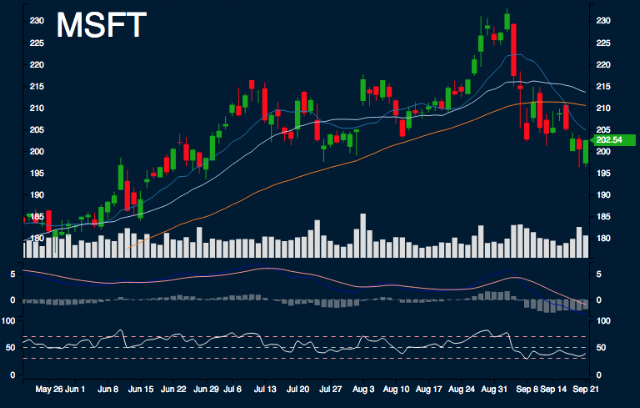#MSFT Report

Sample Size: 7; Percent Error: 10%

## Current Trend Performance

MSFT is -14.37% from monthly high, 3.07% from monthly low, -1.12% from 10 day simple moving average, -5.16% from 20 day simple moving average, and -3.78% from 50 day simple moving average.

## Forecast Ranges

Day 1:
The highs are 203.38, the lows are 196.25, and the median is 199.81. 14.29% to decrease between -4.68% - -2.34%. 42.86% to decrease between -2.34% - 0%. 42.86% to increase between 0% - 2.34%.
Day 2:
The highs are 206.53, the lows are 198.93, and the median is 202.73. 57.14% to decrease between -2.34% - 0%. 42.86% to increase between 0% - 2.34%.
Day 3:
The highs are 204.68, the lows are 200.08, and the median is 202.38. 57.14% to decrease between -2.34% - 0%. 42.86% to increase between 0% - 2.34%.
Day 4:
The highs are 204.79, the lows are 198.1, and the median is 201.44. 57.14% to decrease between -2.34% - 0%. 42.86% to increase between 0% - 2.34%.
Day 5:
The highs are 208.67, the lows are 194.51, and the median is 201.59. 14.29% to decrease between -4.68% - -2.34%. 71.43% to decrease between -2.34% - 0%. 14.29% to increase between 2.34% - 4.68%.
Day 6:
The highs are 208.78, the lows are 195.19, and the median is 201.99. 14.29% to decrease between -4.68% - -2.34%. 57.14% to decrease between -2.34% - 0%. 14.29% to increase between 0% - 2.34%. 14.29% to increase between 2.34% - 4.68%.
Day 7:
The highs are 205.77, the lows are 199.23, and the median is 202.5. 57.14% to decrease between -2.34% - 0%. 42.86% to increase between 0% - 2.34%.

## Summary

SMA: Simple Moving Average

In next 5 days, there is possible support range between 197.8 and 198.48. There is possible resistance range between 203.22 and 207.28. Areas of uncertainty are beyond 197.14 and beyond 211.44. Minimum movement range is 2.34%.

Research conducted with Nforecast.

This is not a recommendation to buy, sell, or hold. This research is for educational, informational, and entertainment purposes. You should not construe any data or information presented by members on this blog or Nforecast LLC as investment, tax, legal, financial or other advice.